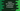# Python set isdisjoint() explanation with an example## Python set isdisjoint()  :

In this tutorial, we will learn about_ isdisjoint()_ method of python set with example._ isdisjoint() method is used to find out if two _sets are disjoint or not. Two sets are called disjoint if they don’t have any common elements.

For example : setA = {1,4,6,8} setB = {2,3,5,7}

Here, setA and setB are disjoint set because no elements are common. Our program will take the inputs for both sets from the user and print out the result if they are disjoint or not.

## Syntax of Python isdisjoint :

The syntax of the isdisjoint method is as below :

``setA.isdisjoint(setB)``

Here, both setA and setB are two python sets.

It takes one parameter. This parameter can be a set or any other iterable. If you pass any other iterable, it will convert that iterable to a set.

This method will return one boolean value. It will return True if the sets are disjoint. Else, it will return False.

## Example Program to show how python isdisjoint works:

``````#1
setA = set()
setB = set()

#2
lengthA = int(input("Enter the size of the first set : "))
lengthB = int(input("Enter the size of the second set : "))

#3
print("\n")
print("For the first set : \n")
for i in range(lengthA):
e = int(input("Enter value {} : ".format(i+1)))

#4
print("\n")
print("Enter values for the second set one by one : \n")
for i in range(lengthB):
e = int(input("Enter value {} : ".format(i+1)))

#5
print("\nYou have entered : ")
print("setA : {} ".format(setA))
print("setB : {} ".format(setB))

#6
if(setA.isdisjoint(setB)):
print("setA and setB are disjoint set")
else:
print("setA and setB are not disjoint set")``````

### Explanation :

The commented numbers in the above program denote the step numbers below :

1. Create two empty sets setA and setB.
2. Read the length of the sets as input from the user and store them in lengthA and lengthB variables.
3. Take the inputs for the first set one by one from the user. Add all values to the first set setA.
4. Similarly, take the inputs for the second set one by one and add all values to the second set setB.
5. Print the values of both sets to the user.
6. Use isdisjoint to check if the sets are disjoint or not. And print out the message accordingly.

## Sample Output :

``````Enter the size of the first set : 3
Enter the size of the second set : 1

For the first set :

Enter value 1 : 2
Enter value 2 : 3
Enter value 3 : 4

Enter values for the second set one by one :

Enter value 1 : 5

You have entered :
setA : {2, 3, 4}
setB : {5}
setA and setB are disjoint set

Enter the size of the first set : 2
Enter the size of the second set : 2

For the first set :

Enter value 1 : 1
Enter value 2 : 2

Enter values for the second set one by one :

Enter value 1 : 2
Enter value 2 : 3

You have entered :
setA : {1, 2}
setB : {2, 3}
setA and setB are not disjoint set``````